Autonomous Driving AI for Donkey Car Garbage Collector

Use a TensorFlow SSD MobileNetV2 DNN on the Raspberry Pi plus Pi Camera to build an autonomous car capable of object detection.

AdvancedFull instructions providedOver 3 days3,898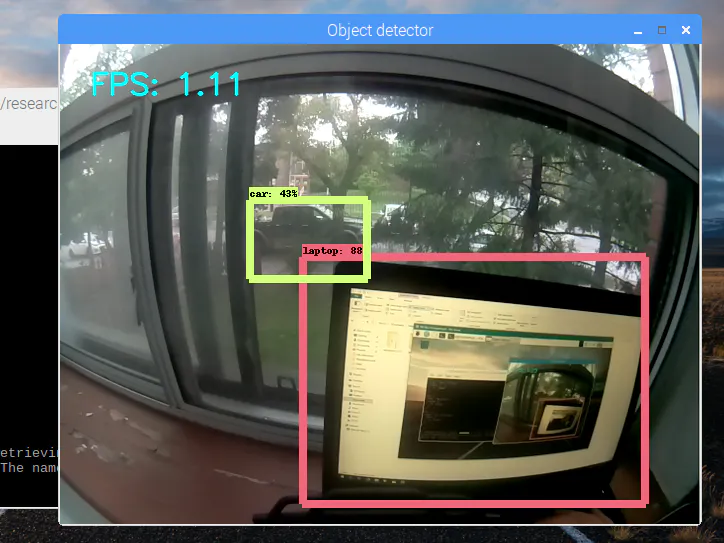Things used in this project

Hardware componentsRaspberry Pi 3 Model B
×1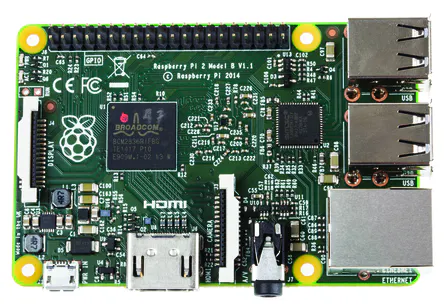Raspberry Pi 2 Model B
×1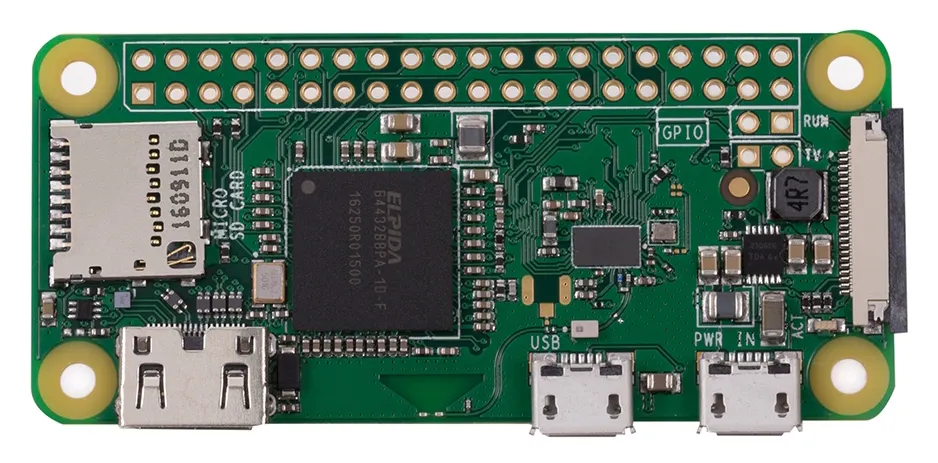Raspberry Pi Zero Wireless
×1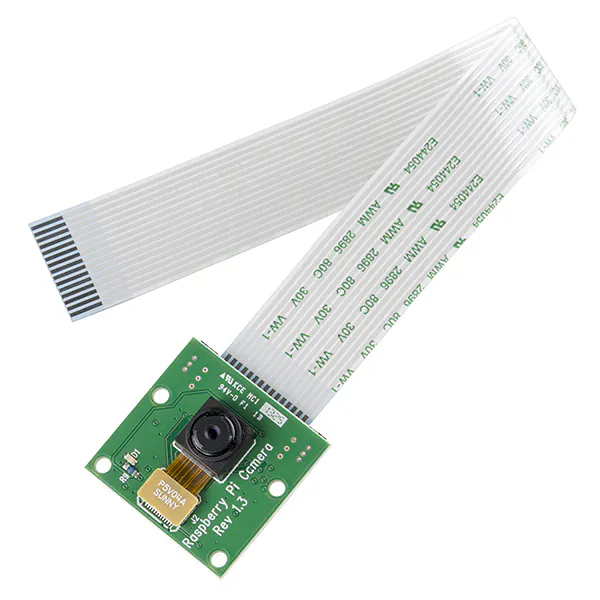Raspberry Pi Camera Module
×1
 DonkeyCar
×1Ultrasonic Sensor - HC-SR04 (Generic)
×1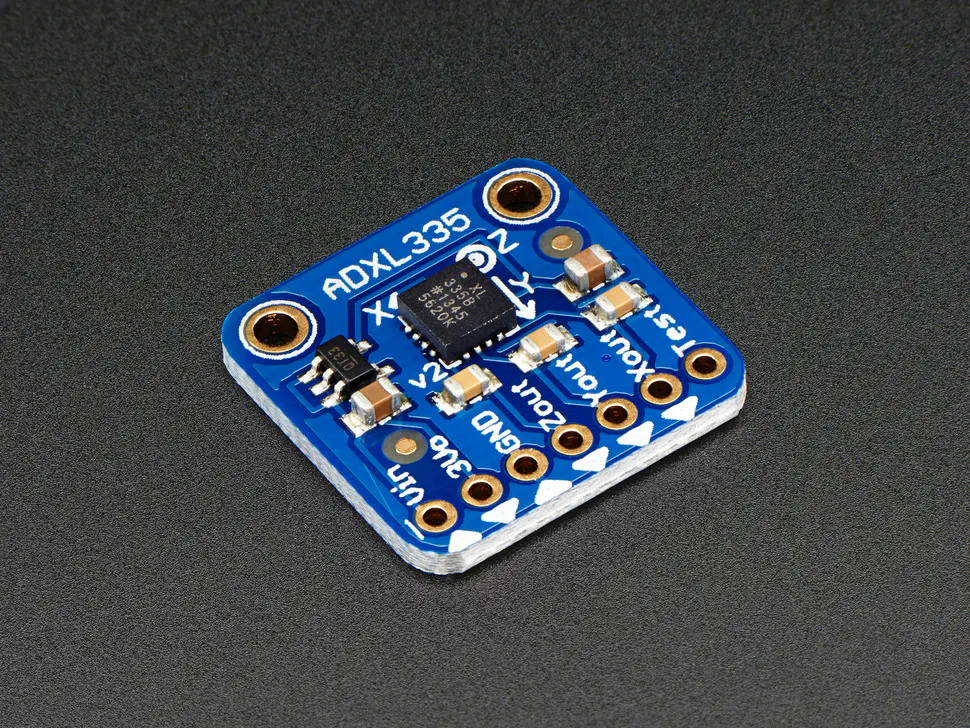×1

Software apps and online services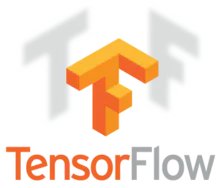TensorFlowOpenCV
 DonkeyCar

Hand tools and fabrication machines

 Screwdriver

Schematics

MPU6050

Connection to MPU6050 IMU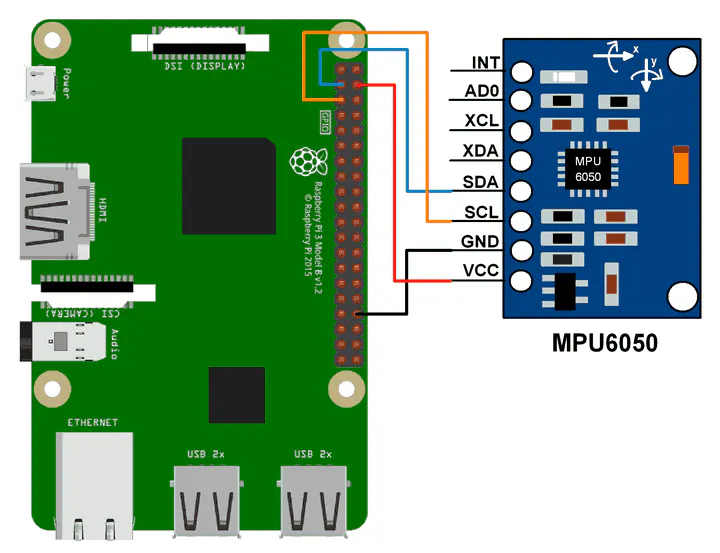Code

IMU code

Python
This is a custom part for the IMU
#!/usr/bin/env python
"""
copyright dhq 2018, GPLV3
"""

from mpu6050 import mpu6050
from time import sleep

sensor = mpu6050(0x68)

class MPU6050():

def run(self,):
accel_data = sensor.get_accel_data()
gyro_data = sensor.get_gyro_data()
temp = sensor.get_temp()
mpu6050pack =    ("x: " + str(accel_data['x'])) + ("y: " + str(accel_data['y'])) + ("z: " + str(accel_data['z'])) + ("gx: " + str(gyro_data['x'])) + ("gy: " + str(gyro_data['y'])) + ("gz: " + str(gyro_data['z'])) + ("Temp: " + str(temp) + " C")
return mpu6050pack

manage.py

Python
Augmented with IMU recording capability..

Issue python manage.py drive to record data.
#!/usr/bin/env python3
"""
Scripts to drive a donkey 2 car and train a model for it.

Usage:
manage.py (drive) [--model=<model>] [--js] [--chaos]
manage.py (train) [--tub=<tub1,tub2,..tubn>]  (--model=<model>) [--base_model=<base_model>] [--no_cache]

Options:
-h --help        Show this screen.
--tub TUBPATHS   List of paths to tubs. Comma separated. Use quotes to use wildcards. ie "~/tubs/*"
--js             Use physical joystick.
--chaos          Add periodic random steering when manually driving
"""
import os
from docopt import docopt

import donkeycar as dk

#import parts
from donkeycar.parts.camera import PiCamera
from donkeycar.parts.transform import Lambda
from donkeycar.parts.keras import KerasCategorical
from donkeycar.parts.actuator import PCA9685, PWMSteering, PWMThrottle
from donkeycar.parts.datastore import TubGroup, TubWriter
from donkeycar.parts.controller import LocalWebController, JoystickController
from donkeycar.parts.clock import Timestamp
from donkeycar.parts.imu import Mpu6050

def drive(cfg, model_path=None, use_joystick=False, use_chaos=False):
"""
Construct a working robotic vehicle from many parts.
Each part runs as a job in the Vehicle loop, calling either
it's run or run_threaded method depending on the constructor flag `threaded`.
All parts are updated one after another at the framerate given in
cfg.DRIVE_LOOP_HZ assuming each part finishes processing in a timely manner.
Parts may have named outputs and inputs. The framework handles passing named outputs
to parts requesting the same named input.
"""

V = dk.vehicle.Vehicle()

imu = Mpu6050()
V.add(imu, outputs=['imu/acl_x', 'imu/acl_y', 'imu/acl_z', 'imu/gyr_x', 'imu/gyr_y', 'imu/gyr_z'], threaded=True)

clock = Timestamp()

cam = PiCamera(resolution=cfg.CAMERA_RESOLUTION)

if use_joystick or cfg.USE_JOYSTICK_AS_DEFAULT:
ctr = JoystickController(max_throttle=cfg.JOYSTICK_MAX_THROTTLE,
steering_scale=cfg.JOYSTICK_STEERING_SCALE,
auto_record_on_throttle=cfg.AUTO_RECORD_ON_THROTTLE)
else:
# This web controller will create a web server that is capable
# of managing steering, throttle, and modes, and more.
ctr = LocalWebController(use_chaos=use_chaos)

inputs=['cam/image_array'],
outputs=['user/angle', 'user/throttle', 'user/mode', 'recording'],

# See if we should even run the pilot module.
# This is only needed because the part run_condition only accepts boolean
def pilot_condition(mode):
if mode == 'user':
return False
else:
return True

pilot_condition_part = Lambda(pilot_condition)
outputs=['run_pilot'])

# Run the pilot if the mode is not user.
kl = KerasCategorical()
if model_path:

outputs=['pilot/angle', 'pilot/throttle'],
run_condition='run_pilot')

# Choose what inputs should change the car.
def drive_mode(mode,
user_angle, user_throttle,
pilot_angle, pilot_throttle):
if mode == 'user':
return user_angle, user_throttle

elif mode == 'local_angle':
return pilot_angle, user_throttle

else:
return pilot_angle, pilot_throttle

drive_mode_part = Lambda(drive_mode)
inputs=['user/mode', 'user/angle', 'user/throttle', 'pilot/angle', 'pilot/throttle'],
outputs=['angle', 'throttle'])

steering_controller = PCA9685(cfg.STEERING_CHANNEL)
steering = PWMSteering(controller=steering_controller,
left_pulse=cfg.STEERING_LEFT_PWM,
right_pulse=cfg.STEERING_RIGHT_PWM)

throttle_controller = PCA9685(cfg.THROTTLE_CHANNEL)
throttle = PWMThrottle(controller=throttle_controller,
max_pulse=cfg.THROTTLE_FORWARD_PWM,
zero_pulse=cfg.THROTTLE_STOPPED_PWM,
min_pulse=cfg.THROTTLE_REVERSE_PWM)

# add tub to save data
inputs = ['cam/image_array', 'user/angle', 'user/throttle', 'user/mode', 'imu/acl_x', 'imu/acl_y', 'imu/acl_z','imu/gyr_x', 'imu/gyr_y', 'imu/gyr_z']
types = ['image_array', 'float', 'float',  'str', 'float', 'float', 'float','float', 'float', 'float']

#multiple tubs
#th = TubHandler(path=cfg.DATA_PATH)
#tub = th.new_tub_writer(inputs=inputs, types=types)

# single tub
tub = TubWriter(path=cfg.TUB_PATH, inputs=inputs, types=types)

# run the vehicle
V.start(rate_hz=cfg.DRIVE_LOOP_HZ, max_loop_count=cfg.MAX_LOOPS)

def train(cfg, tub_names, new_model_path, base_model_path=None ):
"""
use the specified data in tub_names to train an artifical neural network
saves the output trained model as model_name
"""
X_keys = ['cam/image_array']
y_keys = ['user/angle', 'user/throttle']
def train_record_transform(record):
""" convert categorical steering to linear and apply image augmentations """
record['user/angle'] = dk.util.data.linear_bin(record['user/angle'])
# TODO add augmentation that doesn't use opencv
return record

def val_record_transform(record):
""" convert categorical steering to linear """
record['user/angle'] = dk.util.data.linear_bin(record['user/angle'])
return record

new_model_path = os.path.expanduser(new_model_path)

kl = KerasCategorical()
if base_model_path is not None:
base_model_path = os.path.expanduser(base_model_path)

print('tub_names', tub_names)
if not tub_names:
tub_names = os.path.join(cfg.DATA_PATH, '*')
tubgroup = TubGroup(tub_names)
train_gen, val_gen = tubgroup.get_train_val_gen(X_keys, y_keys,
train_record_transform=train_record_transform,
val_record_transform=val_record_transform,
batch_size=cfg.BATCH_SIZE,
train_frac=cfg.TRAIN_TEST_SPLIT)

total_records = len(tubgroup.df)
total_train = int(total_records * cfg.TRAIN_TEST_SPLIT)
total_val = total_records - total_train
print('train: %d, validation: %d' % (total_train, total_val))
steps_per_epoch = total_train // cfg.BATCH_SIZE
print('steps_per_epoch', steps_per_epoch)

kl.train(train_gen,
val_gen,
saved_model_path=new_model_path,
steps=steps_per_epoch,
train_split=cfg.TRAIN_TEST_SPLIT)

if __name__ == '__main__':
args = docopt(__doc__)

if args['drive']:
drive(cfg, model_path = args['--model'], use_joystick=args['--js'], use_chaos=args['--chaos'])

elif args['train']:
tub = args['--tub']
new_model_path = args['--model']
base_model_path = args['--base_model']
cache = not args['--no_cache']
train(cfg, tub, new_model_path, base_model_path)

meta.json

JavaScript
Modified JSON format data for storing MPU6050 values
{"inputs": ["cam/image_array", "user/angle", "user/throttle", "user/mode", "imu/acl_x", "imu/acl_y", "imu/acl_z","imu/gyr_x", "imu/gyr_y", "imu/gyr_z"], "types": ["image_array", "float", "float", "str", "float", "float", "float","float", "float", "float"]}

config.py

Python
Uses calibrated values for steering and throtling.
"""
CAR CONFIG

This file is read by your car application's manage.py script to change the car
performance.

EXMAPLE
-----------
import dk
print(cfg.CAMERA_RESOLUTION)

"""

import os

#PATHS
CAR_PATH = PACKAGE_PATH = os.path.dirname(os.path.realpath(__file__))
DATA_PATH = os.path.join(CAR_PATH, 'data')
MODELS_PATH = os.path.join(CAR_PATH, 'models')

#VEHICLE
DRIVE_LOOP_HZ = 20
MAX_LOOPS = 100000

#CAMERA
CAMERA_RESOLUTION = (120, 160) #(height, width)
CAMERA_FRAMERATE = DRIVE_LOOP_HZ

#STEERING
STEERING_CHANNEL = 1
STEERING_LEFT_PWM = 420
STEERING_RIGHT_PWM = 300

#THROTTLE
THROTTLE_CHANNEL = 0
THROTTLE_FORWARD_PWM = 400
THROTTLE_STOPPED_PWM = 360
THROTTLE_REVERSE_PWM = 310

#TRAINING
BATCH_SIZE = 128
TRAIN_TEST_SPLIT = 0.8

#JOYSTICK
USE_JOYSTICK_AS_DEFAULT = False
JOYSTICK_MAX_THROTTLE = 0.25
JOYSTICK_STEERING_SCALE = 1.0
AUTO_RECORD_ON_THROTTLE = True

TUB_PATH = os.path.join(CAR_PATH, 'tub') # if using a single tub

#ROPE.DONKEYCAR.COM
ROPE_TOKEN="GET A TOKEN AT ROPE.DONKEYCAR.COM"

ObjecDetectionDonkeyCar.py

Python
Code for object detection using Tensorflow and OpenCV.
from virtualenv issue:
python ObjecDetectionDonkeyCar.py
######## Picamera Object Detection Using Tensorflow Classifier #########
#
# Author dhq 		 # Date: 9/12/18
# removed usb camera

# This program uses a TensorFlow classifier to perform object detection.
# It loads the classifier uses it to perform object detection on a Picamera feed.
# It draws boxes and scores around the objects of interest in each frame from the Picamera.

## Based on code from Evan Juras # Date: 4/15/1
## Some of the code is copied from Google's example at
## https://github.com/tensorflow/models/blob/master/research/object_detection/object_detection_tutorial.ipynb
## and some is copied from Dat Tran's example at
## https://github.com/datitran/object_detector_app/blob/master/object_detection_app.py

# Import packages
import os
import cv2
import numpy as np
from picamera.array import PiRGBArray
from picamera import PiCamera
import tensorflow as tf
import argparse
import sys

# Set up camera constants
IM_WIDTH = 160
IM_HEIGHT = 128

# This is needed since the working directory is the object_detection folder.
sys.path.append('..')

# Import utilites
from utils import label_map_util
from utils import visualization_utils as vis_util

# Name of the directory containing the object detection module we're using
MODEL_NAME = 'ssdlite_mobilenet_v2_coco_2018_05_09'

# Grab path to current working directory
CWD_PATH = os.getcwd()

# Path to frozen detection graph .pb file, which contains the model that is used
# for object detection.
PATH_TO_CKPT = os.path.join(CWD_PATH,MODEL_NAME,'frozen_inference_graph.pb')

# Path to label map file
PATH_TO_LABELS = os.path.join(CWD_PATH,'data','mscoco_label_map.pbtxt')

# Number of classes the object detector can identify
NUM_CLASSES = 90

## Load the label map.
# Label maps map indices to category names, so that when the convolution
# network predicts `5`, we know that this corresponds to `airplane`.
# Here we use internal utility functions, but anything that returns a
# dictionary mapping integers to appropriate string labels would be fine
categories = label_map_util.convert_label_map_to_categories(label_map, max_num_classes=NUM_CLASSES, use_display_name=True)
category_index = label_map_util.create_category_index(categories)

# Load the Tensorflow model into memory.
detection_graph = tf.Graph()
with detection_graph.as_default():
od_graph_def = tf.GraphDef()
with tf.gfile.GFile(PATH_TO_CKPT, 'rb') as fid:
od_graph_def.ParseFromString(serialized_graph)
tf.import_graph_def(od_graph_def, name='')

sess = tf.Session(graph=detection_graph)

# Define input and output tensors (i.e. data) for the object detection classifier

# Input tensor is the image
image_tensor = detection_graph.get_tensor_by_name('image_tensor:0')

# Output tensors are the detection boxes, scores, and classes
# Each box represents a part of the image where a particular object was detected
detection_boxes = detection_graph.get_tensor_by_name('detection_boxes:0')

# Each score represents level of confidence for each of the objects.
# The score is shown on the result image, together with the class label.
detection_scores = detection_graph.get_tensor_by_name('detection_scores:0')
detection_classes = detection_graph.get_tensor_by_name('detection_classes:0')

# Number of objects detected
num_detections = detection_graph.get_tensor_by_name('num_detections:0')

# Initialize frame rate calculation
frame_rate_calc = 1
freq = cv2.getTickFrequency()
font = cv2.FONT_HERSHEY_SIMPLEX

# Initialize camera and perform object detection.

# Initialize Picamera and grab reference to the raw capture
camera = PiCamera()
camera.resolution = (IM_WIDTH,IM_HEIGHT)
camera.framerate = 10
rawCapture = PiRGBArray(camera, size=(IM_WIDTH,IM_HEIGHT))
rawCapture.truncate(0)

#To save video file

#path = ('/home/pi/Documents/Obje.avi')
#fourcc = cv2.VideoWriter_fourcc(*'XVID')
#video_writer = cv2.VideoWriter(path,-1, 1, (160,128), True)
# if not video_writer :
# print ("!!! Failed VideoWriter: invalid parameters")
# sys.exit(1)

#camera.start_recording('/home/pi/Documents/Obje.h264')

for frame1 in camera.capture_continuous(rawCapture, format="bgr",use_video_port=True):

t1 = cv2.getTickCount()

# Acquire frame and expand frame dimensions to have shape: [1, None, None, 3]
# i.e. a single-column array, where each item in the column has the pixel RGB value
frame = frame1.array
frame.setflags(write=1)
frame_expanded = np.expand_dims(frame, axis=0)

# Perform the actual detection by running the model with the image as input
(boxes, scores, classes, num) = sess.run(
[detection_boxes, detection_scores, detection_classes, num_detections],
feed_dict={image_tensor: frame_expanded})

# Draw the results of the detection (aka 'visulaize the results')
vis_util.visualize_boxes_and_labels_on_image_array(
frame,
np.squeeze(boxes),
np.squeeze(classes).astype(np.int32),
np.squeeze(scores),
category_index,
use_normalized_coordinates=True,
line_thickness=8,
min_score_thresh=0.40)

cv2.putText(frame,"FPS: {0:.2f}".format(frame_rate_calc),(30,50),font,1,(255,255,0),2,cv2.LINE_AA)

# All the results have been drawn on the frame, so it's time to display it.
cv2.imshow('Object detector', frame)

#video_writer.write(frame)

t2 = cv2.getTickCount()
time1 = (t2-t1)/freq
frame_rate_calc = 1/time1

# Press 'q' to quit
if cv2.waitKey(1) == ord('q'):
break

rawCapture.truncate(0)

camera.close()
#camera.stop_recording()

cv2.destroyAllWindows()
#video_writer.release()

Keras_VGG_test.py

Python
Python Keras_VGG_test.py
from keras.preprocessing.image import load_img
from keras.preprocessing.image import img_to_array
from keras.applications.vgg16 import preprocess_input
from keras.applications.vgg16 import decode_predictions
from keras.applications.vgg16 import VGG16

# let's keep our keras backend tensorflow quiet
import os
os.environ['TF_CPP_MIN_LOG_LEVEL']='3'
# for testing on CPU
#os.environ['CUDA_VISIBLE_DEVICES'] = ''

# load the model
model = VGG16()
# load an image from file
image = load_img('garbage_bin5.jpg', target_size=(224, 224))
# convert the image pixels to a numpy array
image = img_to_array(image)
# reshape data for the model
image = image.reshape((1, image.shape, image.shape, image.shape))
# prepare the image for the VGG model
image = preprocess_input(image)
# predict the probability across all output classes
yhat = model.predict(image)
# convert the probabilities to class labels
label = decode_predictions(yhat)
# retrieve the most likely result, e.g. highest probability
label = label
# print the classification
print('%s (%.2f%%)' % (label, label*100))

Fixes an issue with KerasCategorical class.

Python
Replace this file with Keras.py on the donkey /parts folder and issue pip install e .
""""
keras.py
functions to run and train autopilots using keras
"""

from tensorflow.python.keras.layers import Input
from tensorflow.python.keras.models import Model, load_model
from tensorflow.python.keras.layers import Convolution2D
from tensorflow.python.keras.layers import Dropout, Flatten, Dense, Cropping2D, Lambda
from tensorflow.python.keras.callbacks import ModelCheckpoint, EarlyStopping

from donkeycar import util

class KerasPilot:

def shutdown(self):
pass

def train(self, train_gen, val_gen,
saved_model_path, epochs=100, steps=100, train_split=0.8,
verbose=1, min_delta=.0005, patience=5, use_early_stop=True):
"""
train_gen: generator that yields an array of images an array of
"""

# checkpoint to save model after each epoch
save_best = ModelCheckpoint(saved_model_path,
monitor='val_loss',
verbose=verbose,
save_best_only=True,
mode='min')

# stop training if the validation error stops improving.
early_stop = EarlyStopping(monitor='val_loss',
min_delta=min_delta,
patience=patience,
verbose=verbose,
mode='auto')

callbacks_list = [save_best]

if use_early_stop:
callbacks_list.append(early_stop)

hist = self.model.fit_generator(
train_gen,
steps_per_epoch=steps,
epochs=epochs,
verbose=1,
validation_data=val_gen,
callbacks=callbacks_list,
validation_steps=steps * (1.0 - train_split) / train_split)
return hist

class KerasCategorical(KerasPilot):
def __init__(self, model=None, *args, **kwargs):
super(KerasCategorical, self).__init__(*args, **kwargs)
if model:
self.model = model
else:
self.model = default_categorical()

def run(self, img_arr):
img_arr = img_arr.reshape((1,) + img_arr.shape)
angle_binned, throttle = self.model.predict(img_arr)
angle_unbinned = util.data.linear_unbin(angle_binned)
return angle_unbinned, throttle

class KerasLinear(KerasPilot):
def __init__(self, model=None, num_outputs=None, *args, **kwargs):
super(KerasLinear, self).__init__(*args, **kwargs)
if model:
self.model = model
elif num_outputs is not None:
self.model = default_n_linear(num_outputs)
else:
self.model = default_linear()

def run(self, img_arr):
img_arr = img_arr.reshape((1,) + img_arr.shape)
outputs = self.model.predict(img_arr)
# print(len(outputs), outputs)
steering = outputs
throttle = outputs
return steering, throttle

def default_categorical():
img_in = Input(shape=(120, 160, 3),
name='img_in')  # First layer, input layer, Shape comes from camera.py resolution, RGB
x = img_in
x = Convolution2D(24, (5, 5), strides=(2, 2), activation='relu')(
x)  # 24 features, 5 pixel x 5 pixel kernel (convolution, feauture) window, 2wx2h stride, relu activation
x = Convolution2D(32, (5, 5), strides=(2, 2), activation='relu')(
x)  # 32 features, 5px5p kernel window, 2wx2h stride, relu activatiion
x = Convolution2D(64, (5, 5), strides=(2, 2), activation='relu')(
x)  # 64 features, 5px5p kernal window, 2wx2h stride, relu
x = Convolution2D(64, (3, 3), strides=(2, 2), activation='relu')(
x)  # 64 features, 3px3p kernal window, 2wx2h stride, relu
x = Convolution2D(64, (3, 3), strides=(1, 1), activation='relu')(
x)  # 64 features, 3px3p kernal window, 1wx1h stride, relu

# Possibly add MaxPooling (will make it less sensitive to position in image).  Camera angle fixed, so may not to be needed

x = Flatten(name='flattened')(x)  # Flatten to 1D (Fully connected)
x = Dense(100, activation='relu')(x)  # Classify the data into 100 features, make all negatives 0
x = Dropout(.1)(x)  # Randomly drop out (turn off) 10% of the neurons (Prevent overfitting)
x = Dense(50, activation='relu')(x)  # Classify the data into 50 features, make all negatives 0
x = Dropout(.1)(x)  # Randomly drop out 10% of the neurons (Prevent overfitting)
# categorical output of the angle
angle_out = Dense(15, activation='softmax', name='angle_out')(
x)  # Connect every input with every output and output 15 hidden units. Use Softmax to give percentage. 15 categories and find best one based off percentage 0.0-1.0

# continous output of throttle
throttle_out = Dense(1, activation='relu', name='throttle_out')(x)  # Reduce to 1 number, Positive number only

model = Model(inputs=[img_in], outputs=[angle_out, throttle_out])
loss={'angle_out': 'categorical_crossentropy',
'throttle_out': 'mean_absolute_error'},
loss_weights={'angle_out': 0.9, 'throttle_out': .01})

return model

from tensorflow.python.keras import backend as K

def linear_unbin_layer(tnsr):
bin = K.constant((2 / 14), dtype='float32')
norm = K.constant(1, dtype='float32')

b = K.cast(K.argmax(tnsr), dtype='float32')
a = b - norm
# print('linear_unbin_layer out: {}'.format(a))
return a

def default_catlin():
"""
Categorial Steering output before linear conversion.
:return:
"""
img_in = Input(shape=(120, 160, 3),
name='img_in')  # First layer, input layer, Shape comes from camera.py resolution, RGB
x = img_in
x = Convolution2D(24, (5, 5), strides=(2, 2), activation='relu')(
x)  # 24 features, 5 pixel x 5 pixel kernel (convolution, feauture) window, 2wx2h stride, relu activation
x = Convolution2D(32, (5, 5), strides=(2, 2), activation='relu')(
x)
x = Convolution2D(64, (5, 5), strides=(2, 2), activation='relu')(
x)  # 64 features, 5px5p kernal window, 2wx2h stride, relu
x = Convolution2D(64, (3, 3), strides=(2, 2), activation='relu')(
x)  # 64 features, 3px3p kernal window, 2wx2h stride, relu
x = Convolution2D(64, (3, 3), strides=(1, 1), activation='relu')(
x)  # 64 features, 3px3p kernal window, 1wx1h stride, relu

# Possibly add MaxPooling (will make it less sensitive to position in image).  Camera angle fixed, so may not to be needed

x = Flatten(name='flattened')(x)  # Flatten to 1D (Fully connected)
x = Dense(100, activation='relu')(x)  # Classify the data into 100 features, make all negatives 0
x = Dropout(.1)(x)  # Randomly drop out (turn off) 10% of the neurons (Prevent overfitting)
x = Dense(50, activation='relu')(x)  # Classify the data into 50 features, make all negatives 0
x = Dropout(.1)(x)  # Randomly drop out 10% of the neurons (Prevent overfitting)
# categorical output of the angle
angle_cat_out = Dense(15, activation='softmax', name='angle_cat_out')(x)
angle_out = Dense(1, activation='sigmoid', name='angle_out')(angle_cat_out)
# angle_out = Lambda(linear_unbin_layer, output_shape=(1,1, ), name='angle_out')(angle_cat_out)

# continuous output of throttle
throttle_out = Dense(1, activation='relu', name='throttle_out')(x)  # Reduce to 1 number, Positive number only

model = Model(inputs=[img_in], outputs=[angle_out, throttle_out])
loss={'angle_out': 'mean_squared_error',
'throttle_out': 'mean_absolute_error'},
loss_weights={'angle_out': 0.9, 'throttle_out': .01})

return model

def default_linear():
img_in = Input(shape=(120, 160, 3), name='img_in')
x = img_in
x = Convolution2D(24, (5, 5), strides=(2, 2), activation='relu')(x)
x = Convolution2D(32, (5, 5), strides=(2, 2), activation='relu')(x)
x = Convolution2D(64, (5, 5), strides=(2, 2), activation='relu')(x)
x = Convolution2D(64, (3, 3), strides=(2, 2), activation='relu')(x)
x = Convolution2D(64, (3, 3), strides=(1, 1), activation='relu')(x)

x = Flatten(name='flattened')(x)
x = Dense(100, activation='linear')(x)
x = Dropout(.1)(x)
x = Dense(50, activation='linear')(x)
x = Dropout(.1)(x)
# categorical output of the angle
angle_out = Dense(1, activation='linear', name='angle_out')(x)

# continous output of throttle
throttle_out = Dense(1, activation='linear', name='throttle_out')(x)

model = Model(inputs=[img_in], outputs=[angle_out, throttle_out])

loss={'angle_out': 'mean_squared_error',
'throttle_out': 'mean_squared_error'},
loss_weights={'angle_out': 0.5, 'throttle_out': .5})

return model

def default_n_linear(num_outputs):
img_in = Input(shape=(120, 160, 3), name='img_in')
x = img_in
x = Cropping2D(cropping=((60, 0), (0, 0)))(x)  # trim 60 pixels off top
x = Lambda(lambda x: x / 127.5 - 1.)(x)  # normalize and re-center
x = Convolution2D(24, (5, 5), strides=(2, 2), activation='relu')(x)
x = Convolution2D(32, (5, 5), strides=(2, 2), activation='relu')(x)
x = Convolution2D(64, (5, 5), strides=(1, 1), activation='relu')(x)
x = Convolution2D(64, (3, 3), strides=(1, 1), activation='relu')(x)
x = Convolution2D(64, (3, 3), strides=(1, 1), activation='relu')(x)

x = Flatten(name='flattened')(x)
x = Dense(100, activation='relu')(x)
x = Dropout(.1)(x)
x = Dense(50, activation='relu')(x)
x = Dropout(.1)(x)

outputs = []

for i in range(num_outputs):
outputs.append(Dense(1, activation='linear', name='n_outputs' + str(i))(x))

model = Model(inputs=[img_in], outputs=outputs)